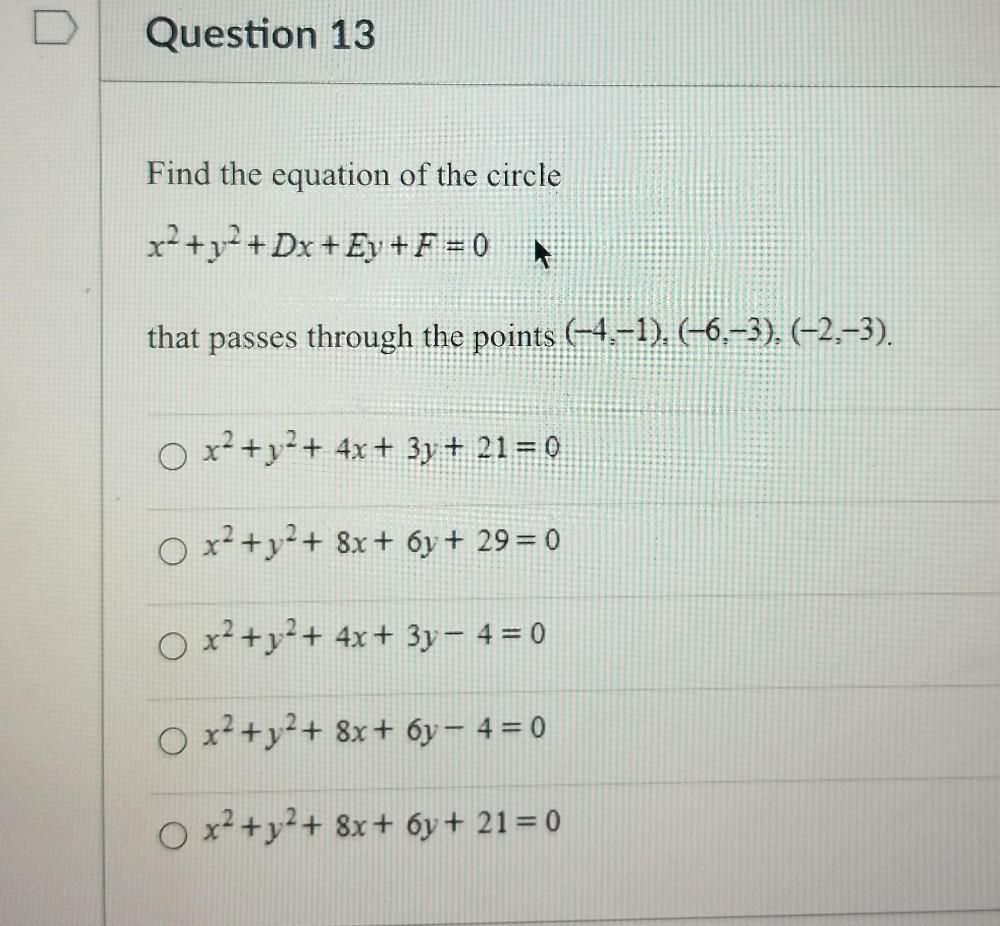Question:

# D Question 13 Find the equation of the circle x2 + y2 + Dx + Ey + F = 0 # that passes through the points (-4,-1). (-6.-3), (-2,-D Question 13 Find the equation of the circle x2 + y2 + Dx + Ey + F = 0 # that passes through the points (-4,-1). (-6.-3), (-2,-3). x2 + y2 + 4x + 3y + 21 = 0 o x2 + y2 + 8x + 6y + 29 = 0 O x2 + y2 + 4x + 3y - 4 = 0 O x2 + y2 + 8x+ 6y - 4 = 0 O x2 + y2 + 8x+ 6y + 21 = 0